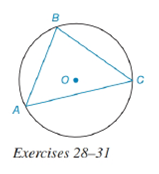Chapter 6.4, Problem 30EElementary Geometry For College St...

7th Edition
Alexander + 2 others
ISBN: 9781337614085

Solutions

Chapter
SectionElementary Geometry For College St...

7th Edition
Alexander + 2 others
ISBN: 9781337614085
Textbook Problem

Given that m A C ⌢ : m B C ⌢ : m A B ⌢ = 4 : 3 : 2 in circle O :a) Which arc is largest?b) Which chord is longest?To determine

(a)

To find:

The largest arc.

Explanation

Given:

mAC:mBC:mAB=4:3:2 in circle O. The figure given below

Theorem Used:

In a circle (or in congruent circles) containing two unequal arcs, the larger arc corresponds to the larger central angle.

Calculation:

Since, mAC:mBC:mAB=4:3:2 and the sum of the arcs is 360°, we get

mAC+mBC+mAB=360°

or 4x+3x+2x=360°

To determine

(b)

To find:

The longest chord.

Still sussing out bartleby?

Check out a sample textbook solution.

See a sample solution

The Solution to Your Study Problems

Bartleby provides explanations to thousands of textbook problems written by our experts, many with advanced degrees!

Get Started

If f(x)=0g(x)11+t3dt, where g(x)=0cosx[1+sin(t2)]dt, find f(/2).

Single Variable Calculus: Early Transcendentals, Volume I

Evaluate each expression: 10+42

Elementary Technical Mathematics

Polynomial Inequalities Solve the inequality. 3. (x 3)(x + 5)(2x + 5) 0

Precalculus: Mathematics for Calculus (Standalone Book)

True or False: Every series must do one of these: converge absolutely, converge conditionally, or diverge.

Study Guide for Stewart's Single Variable Calculus: Early Transcendentals, 8th

True or False: If F is conservative, then curl F = 0.

Study Guide for Stewart's Multivariable Calculus, 8th